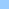# recursion

recursion and an example
recursionaddBit ```>>>> What exactly is the definition of recursion? Is it simply being caught in an infinite repeat loop <<<< I don't think so. >>>> Is it, from the standpoint of logic, necessarily tautological <<<< not really In short, I'm wondering what the relations between recursion, repetition, redundancy, truth, and self-reference are. A ponderous matter, I know, but I'd be curious to know if any of you have thoughts on it. keith seward <<<< Keith, I'm self taught myself but as I understood recursions the clou of it is to design a function in a way that is does just one "repeat" of a variable/unknown amount of "repeats" and then let it decide via the conditional whether it is done or it should call itself once again. On a simple example, very basic in assembler but not so in lingo: add 1 to a byte of n bit. If n = 3 the sequence of results could look like this: [0, 0, 0] [1, 0, 0] [0, 1, 0] [1, 1, 0] [0, 0, 1] [1, 0, 1] [0, 1, 1] [1, 1, 1] The logic is simple: If the digit to the left is 0 then make it 1, done. If it is 1 then make it 0, go to the next digit and (do the same with the next digit) I think there are other solutions to this based on repeat loops but when I encountered a problem which needed an addBit function I found it the most logical and easiest way to make the function call itself until done. on addBit LBits,digit -- lsb is on the left here if voidP(digit) then set digit = 1 -- some ritual error checking first if not listP(LBits) or digit < 1 then return "bad input" set Bit.Cnt = Count(LBits) if digit > Bit.Cnt then return -- the fun starts here set test = getat(LBits,Digit) if not test then setat LBits,digit,TRUE else setat LBits,digit,FALSE set Digit=Digit + 1 if Digit <= Bit.Cnt then addBit(LBits,Digit) end if end if -- this line is unnecessary since we deal with a list -- however, added for convenience of MessWin testing return LBits end ```Home shock + cgi Bits 'n pieces Director Lingo Shocklets Contact

pageID=l_recursion